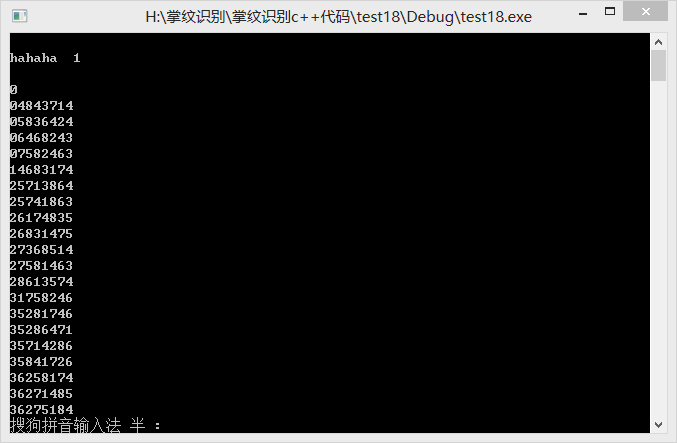record 未被赋值的情况下，前后两次查看，值不一样，运行结果的前两行即是record，一次是1，一次是0

`````` #include<stdio.h>
#include<stdlib.h>
int control={0};
int record={0};
int count=0;
int floor=1;
int tmp;
int p;
void setControlArea(int rowid,int colid){
int i,j;
for(i=rowid+1;i<8;i++) control[i][colid]++;
for(i=rowid+1,j=colid+1;i<8,j<8;i++,j++) control[i][j]++;
for(i=rowid+1,j=colid-1;i>=0,j>=0;i++,j--) control[i][j]++; //只管下面两条线
}
void releaseControlArea(int rowid,int colid){
int i,j;
for(i=rowid+1;i<8;i++) control[i][colid]--;
for(i=rowid+1,j=colid+1;i<8,j<8;i++,j++) control[i][j]--;
for(i=rowid+1,j=colid-1;i>=0,j>=0;i++,j--) control[i][j]--; //只管下面两条线
}
//递归解法
void find(){
int j;
if(floor==8){
for(j=0;j<8;j++){
if(control[j]==0) {
tmp=j+1;
for(p=0;p<8;p++){
record[count][p]=tmp[p];
if(count==0&&p==0) printf("\nhahaha  %d\n",record[count][p]);
}
count++;
break;}
}
floor--;
return;
}
else if(floor==1){
for(j=0;j<8;j++){
tmp=j+1;
setControlArea(0,j);
floor++;
find();
releaseControlArea(0,j);
}
floor--;
return;
}
else{
for(j=0;j<8;j++){
if(control[floor-1][j]==0){
tmp[floor-1]=j+1;
setControlArea(floor-1,j);
floor++;
find();
releaseControlArea(floor-1,j);
}
}
floor--;
}
}
int main(){
int i,j;
find();
printf("\n%d\n",record);
for(i=0;i<count;i++){
for(j=0;j<8;j++){
printf("%d",record[i][j]);
}
printf("\n");
}
printf("%d",count);
system("pause");
}
``````1个回答

record 要赋初始值，否则可以会导致一些莫名其妙的问题，因为里的数据可能是随机的yuexia_wrp 谢谢，解决了，是因为control数组和record数组的空间紧挨着，我在对control数组操作时越界了，导致record中前5行内容被改变了。。。
5 年多之前 回复